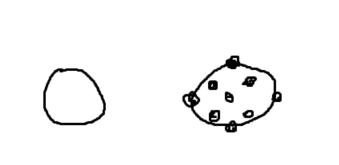# Help making automated parts based on size of part

How would i based on the size of a part’s X,Y,Z make parts inside it at certain points in a radius of the center point diagram hereobviously in 3d not just in 1 line of the part

2 Likes

I’m not sure what you mean, you want to size a sphere based on the distance of one part from the other?

``````local p1 = workspace.Part1
local distancePart = workspace.DistancePart
local p3 = workspace.Part3

local distance = (p1.Position - distancePart.Position).Magnitude
p3.Size = Vector3.new(distance,distance,distance)
``````
1 Like

no let me make something by hand in studio give me a moment

2 Likes

Here’s something I made a long time ago. It could probably be improved and would need to be modified to work in different situations.

``````local count = 3
local size = 1
local off = 1.2
local dis = math.pi*2*size/count
local pos = CFrame.new(0,2,0)
function gen(t)
local o = Instance.new('Part')
o.Name = 'Point'
o.Anchored = true
o.Size = Vector3.new(1,1,1)
o.Shape = t
return o
end
for i=0,count-1 do
local cf = pos*CFrame.Angles(0,dis*i,0)*CFrame.new(0,0,-size*off)
local o = gen(Enum.PartType.Block)
o.CFrame = cf
o.Parent = workspace
end
``````
2 Likes

i will check that out but in response to @7z99this is what im trying to acheive but automated

2 Likes

Oh, I see.

Here’s something quick that could probably be improved upon but it’s just a proof of concept ig.

``````local part = workspace.Part
local size = part.Size

local p1 = Instance.new('Part')
p1.Parent = part
p1.Position = part.Position
p1.Size = Vector3.new(1,1,1)
p1.Anchored = true
for i = -1,1, 2 do
local currentPart = Instance.new('Part')
currentPart.Parent = part
currentPart.Position = p1.Position + Vector3.new(size.X / 2 * i,0,0)
currentPart.Anchored = true
end
for i = -1,1, 2 do
local currentPart = Instance.new('Part')
currentPart.Parent = part
currentPart.Position = p1.Position + Vector3.new(0,size.Y / 2 * i,0)
currentPart.Anchored = true
end
for i = -1,1, 2 do
local currentPart = Instance.new('Part')
currentPart.Parent = part
currentPart.Position = p1.Position + Vector3.new(0,0,size.Z / 2 * i)
currentPart.Anchored = true
end
``````
2 Likes

Try this script I made that uses trig

``````local sphere = script.Parent

for i = 0, 360, 90 do -- iterates through a whole circle of degrees. like 0, 90, 180, 270, 360
local newPart = Instance.new("Part") -- creates the ball for the x axis
newPart.Anchored = true
newPart.Parent = sphere
newPart.Shape = "Ball"
newPart.Size = Vector3.new(1,1,1)
newPart.Color = Color3.fromRGB(85, 255, 0)
local degreeAngle = math.rad(i) -- turns the degrees of my for loop into radians which is what roblox uses for its trig functions